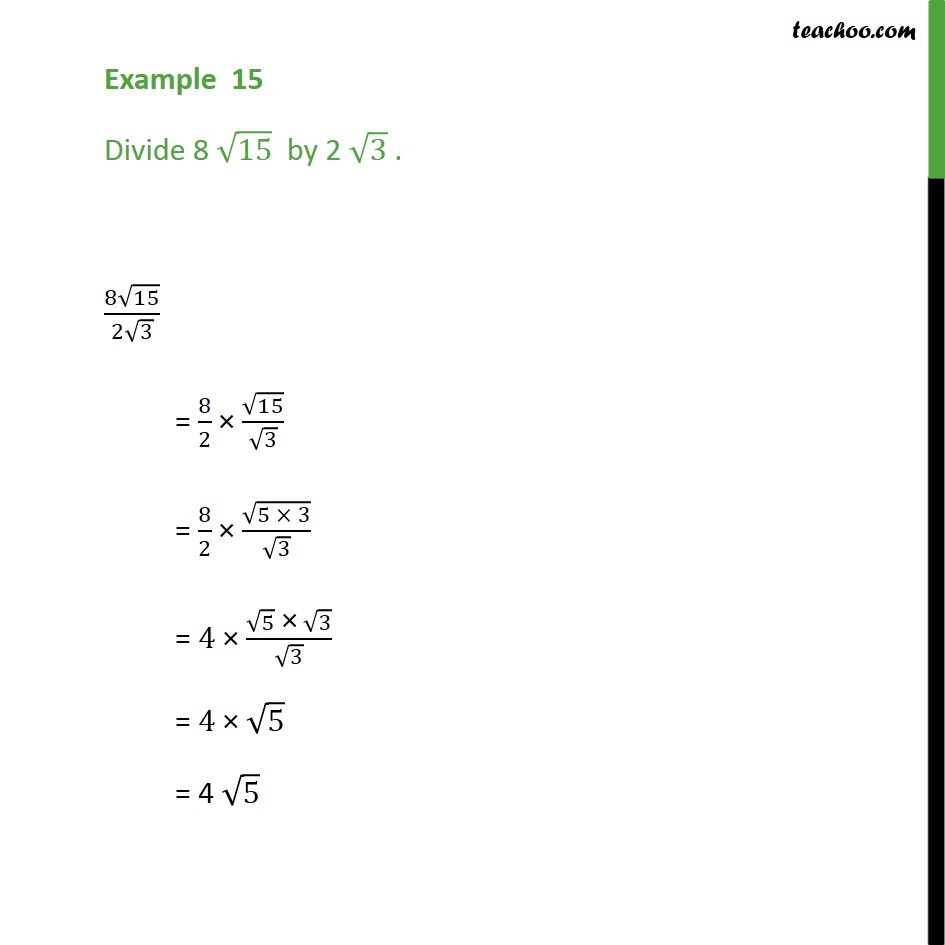1. Chapter 1 Class 9 Number Systems (Term 1)
2. Concept wise
3. Simplifying real numbers

Transcript

Example 15 Divide 8 √15 by 2 √3 . Since both numbers have root We divide integers separately, and roots separately Let's check the video as well (8√15)/(2√3) = 8/2 × √15/√3 = 8/2 × √(5 × 3)/√3 = 4 × (√5 " ×" √3)/√3 = 4 × √5 = 4 √5

Simplifying real numbers